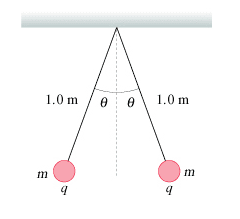# Find the distance between the q charges

## Homework Statement

Two 3.2 g point charges on 1.0-m-long threads repel each other after being equally charged, as shown in the figure.(https://session.masteringphysics.com/problemAsset/1384363/9/25_P59_sf=2.jpg)[Moderator note: Added back the Problem Statement header and inserted the figure to make it visible without following a link]
Assume that θ = 16 ∘.

- Although this problem is asking for q, I am just curious in knowing how to find the distance between the two charges. I tried using law of sines, but can you assume that they are directly across from each other which would be forming a triangle?

## The Attempt at a Solution

- using law of sines i found the distance to be .55 m[/B]

Last edited by a moderator:

haruspex
Homework Helper
Gold Member
2020 Award
can you assume that they are directly across from each other
The two threads are the same length, so you have an isosceles triangle. The common mass centre will be half way between the masses. Where must that be in relation to the support?

First of all,
Use the proper format of Homework Questions :

## The Attempt at a Solution

[/B][/B]

It helps people to understand what is the problem and what you tried to do clearly. First two are absent here.

Now, from what I have understood, there are two forces acting on the the charges in different direction - gravitational force and electrostatic force. Using the force components acting on the string, you can easily get the distance between the charges.